# OpenCV-Python 系列 三十 | 模板匹配

### 目标

– 使用模板匹配在图像中查找对象
– 你将看到以下功能：cv.matchTemplate()，cv.minMaxLoc()

### 理论

OpenCV中实现了几种比较方法。（您可以检查文档以了解更多详细信息）。它返回一个灰度图像，其中每个像素表示该像素的邻域与模板匹配的程度。

### OpenCV中的模板匹配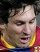import cv2 as cv
import numpy as np
from matplotlib import pyplot as plt
img2 = img.copy()
w, h = template.shape[::-1]
# 列表中所有的6种比较方法
methods = ['cv.TM_CCOEFF', 'cv.TM_CCOEFF_NORMED', 'cv.TM_CCORR',
'cv.TM_CCORR_NORMED', 'cv.TM_SQDIFF', 'cv.TM_SQDIFF_NORMED']
for meth in methods:
img = img2.copy()
method = eval(meth)
# 应用模板匹配
res = cv.matchTemplate(img,template,method)
min_val, max_val, min_loc, max_loc = cv.minMaxLoc(res)
# 如果方法是TM_SQDIFF或TM_SQDIFF_NORMED，则取最小值
if method in [cv.TM_SQDIFF, cv.TM_SQDIFF_NORMED]:
top_left = min_loc
else:
top_left = max_loc
bottom_right = (top_left + w, top_left + h)
cv.rectangle(img,top_left, bottom_right, 255, 2)
plt.subplot(121),plt.imshow(res,cmap = 'gray')
plt.title('Matching Result'), plt.xticks([]), plt.yticks([])
plt.subplot(122),plt.imshow(img,cmap = 'gray')
plt.title('Detected Point'), plt.xticks([]), plt.yticks([])
plt.suptitle(meth)
plt.show()


• cv.TM_CCOEFF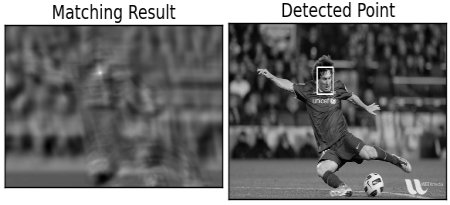• cv.TM_CCOEFF_NORMED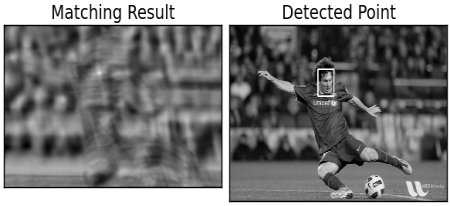• cv.TM_CCORR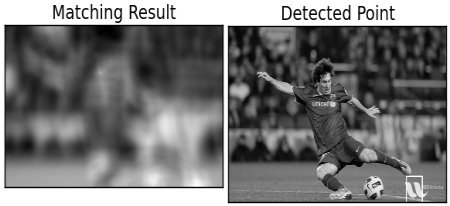• cv.TM_CCORR_NORMED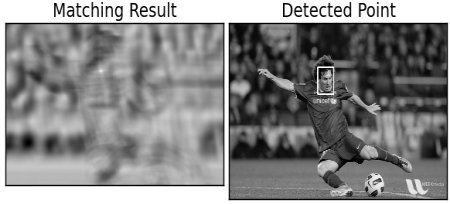• cv.TM_SQDIFF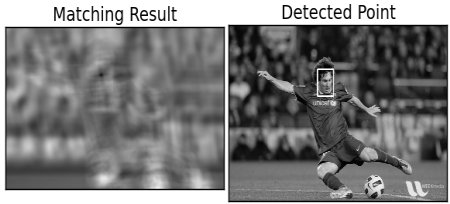• cv.TM_SQDIFF_NORMED### 多对象的模板匹配

import cv2 as cv
import numpy as np
from matplotlib import pyplot as plt
img_gray = cv.cvtColor(img_rgb, cv.COLOR_BGR2GRAY)
w, h = template.shape[::-1]
res = cv.matchTemplate(img_gray,template,cv.TM_CCOEFF_NORMED)
threshold = 0.8
loc = np.where( res >= threshold)
for pt in zip(*loc[::-1]):
cv.rectangle(img_rgb, pt, (pt + w, pt + h), (0,0,255), 2)
cv.imwrite('res.png',img_rgb)